Case Based Questions (MCQ)

Chapter 3 Class 12 Matrices
Serial order wise

## A manufacture produces three stationery products Pencil, Eraser and Sharpener which he sells in two markets. Annual sales are indicated below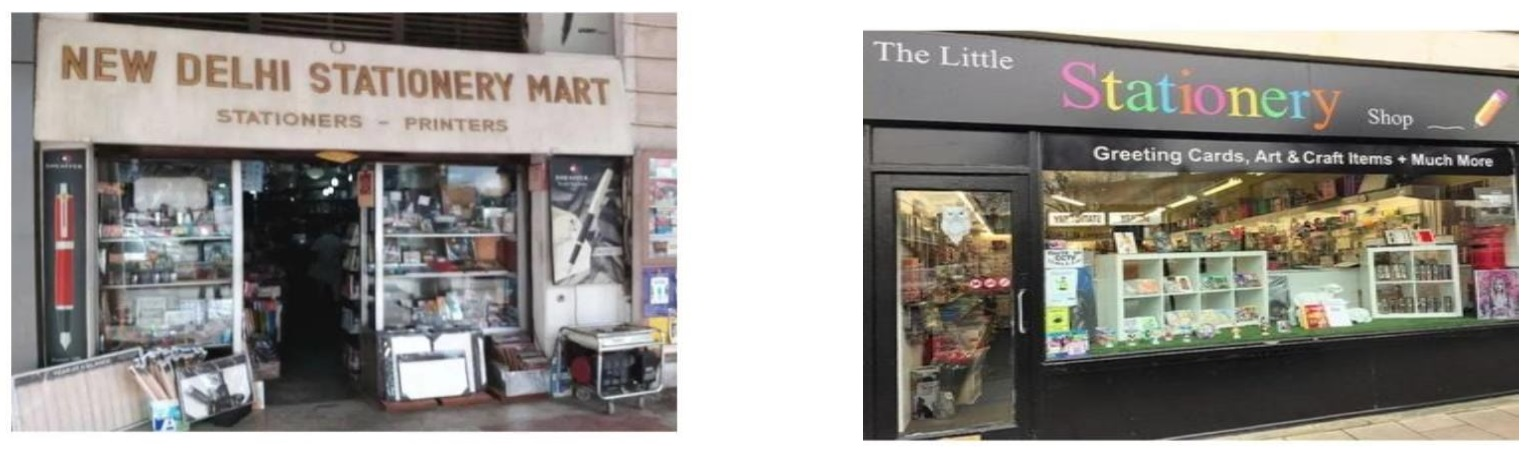Market Products (in numbers) Pencil Eraser Sharpener A 10,000 2000 18,000 B 6,000 20,000 8,000

## If the unit Sale price of Pencil, Eraser and Sharpener are Rs. 2.50, Rs. 1.50 and Rs. 1.00 respectively, and unit cost of the above three commodities are Rs. 2.00, Rs. 1.00 and Rs. 0.50 respectively, then, Based on the above information answer the following: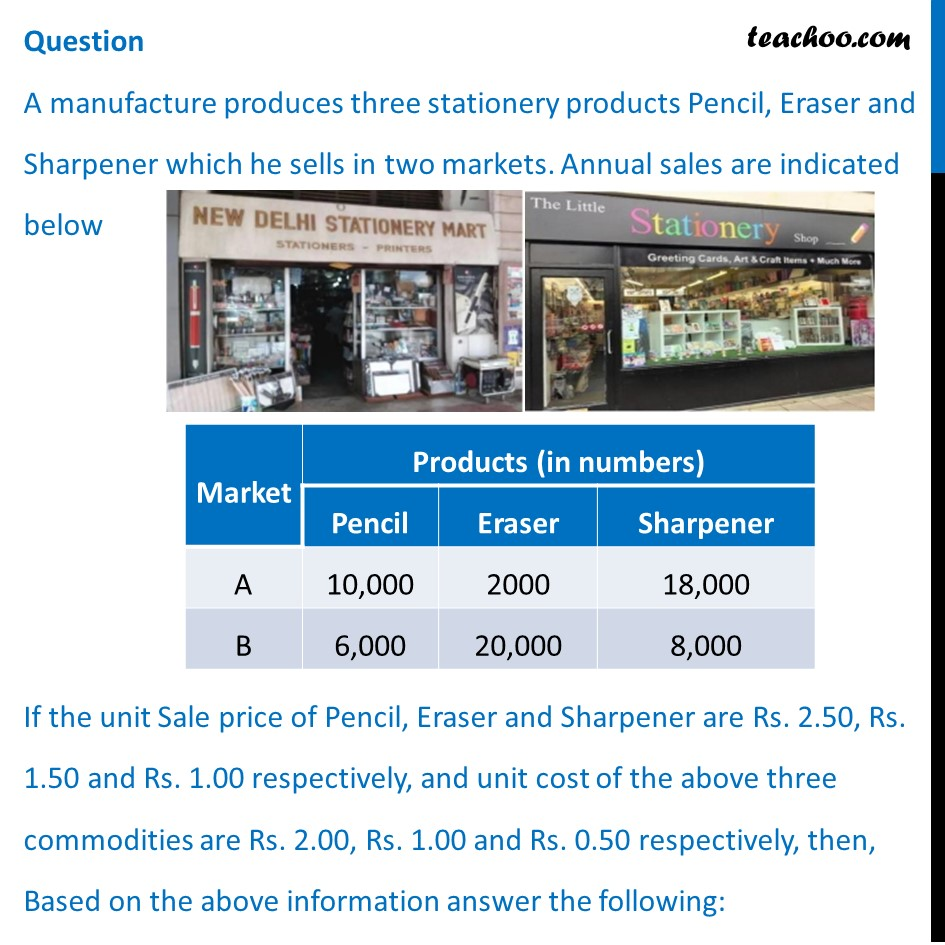## (a) Rs. 64,000 (b) Rs. 60,400 (c) Rs. 46,000 (d) Rs. 40,600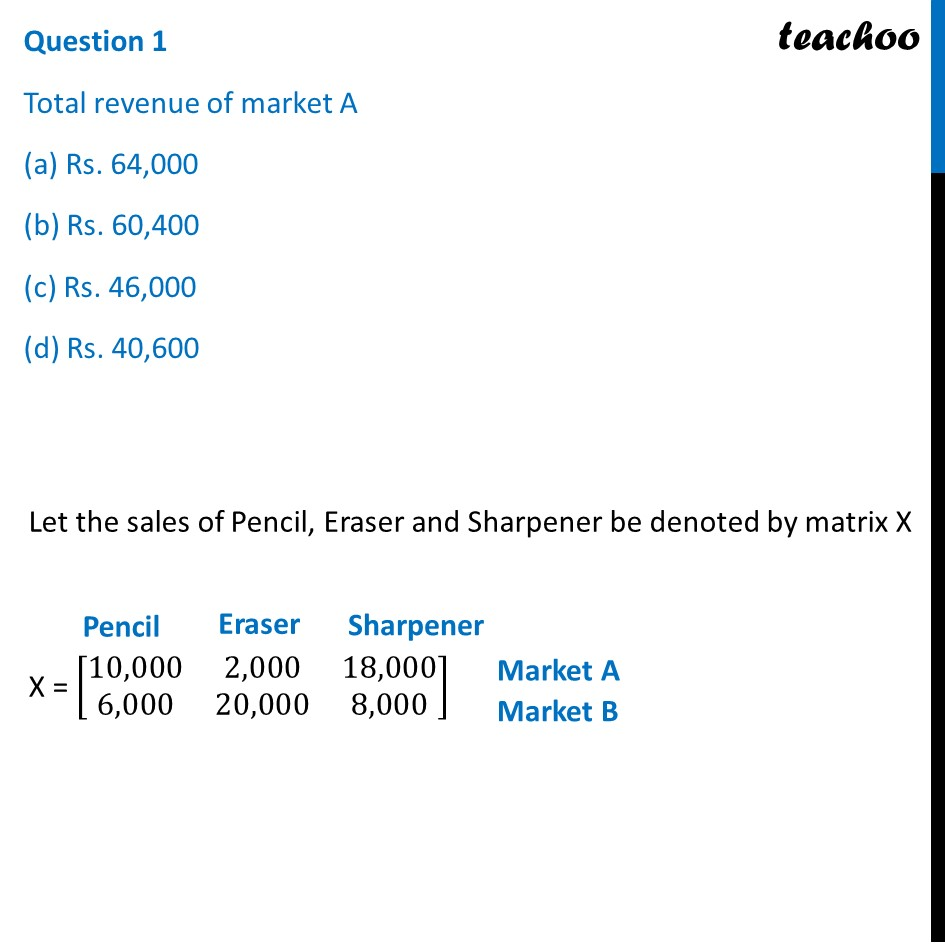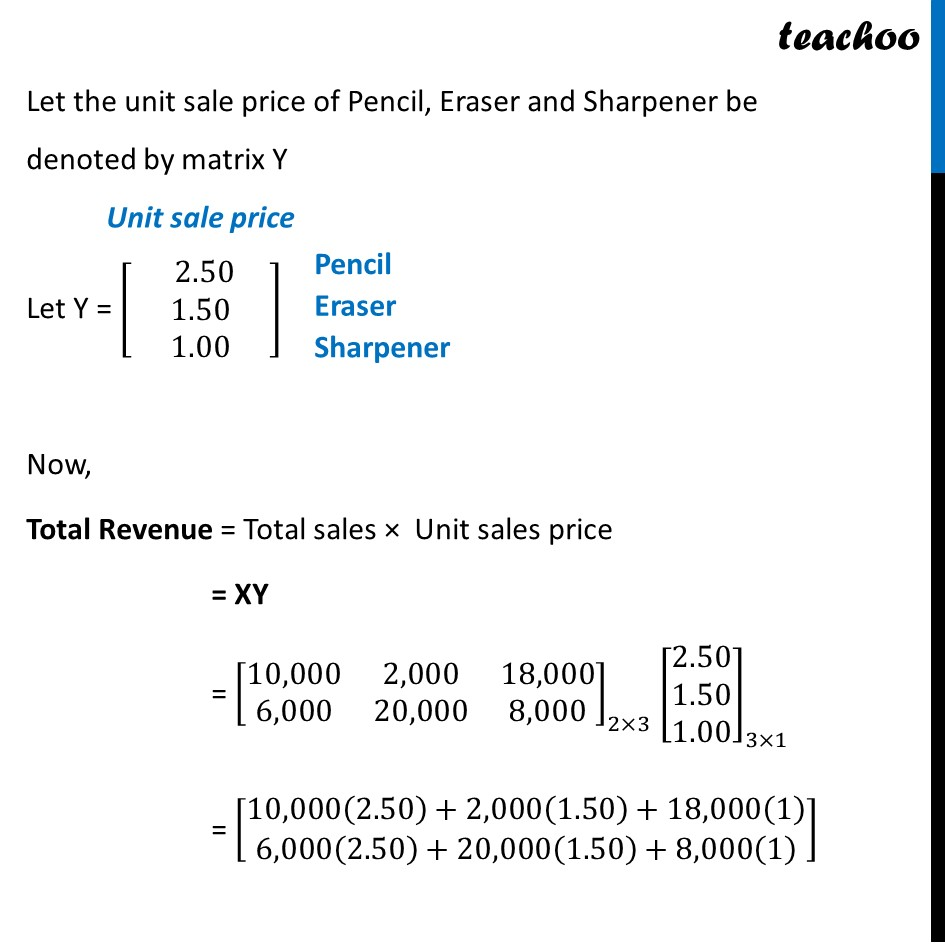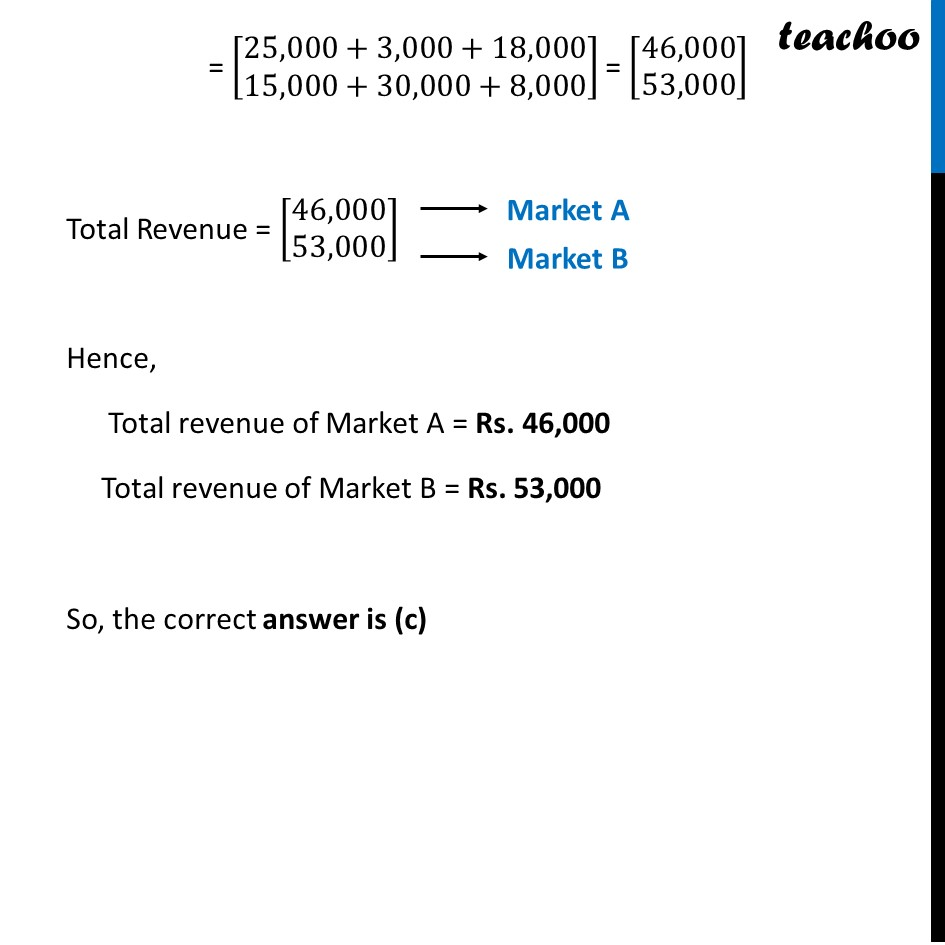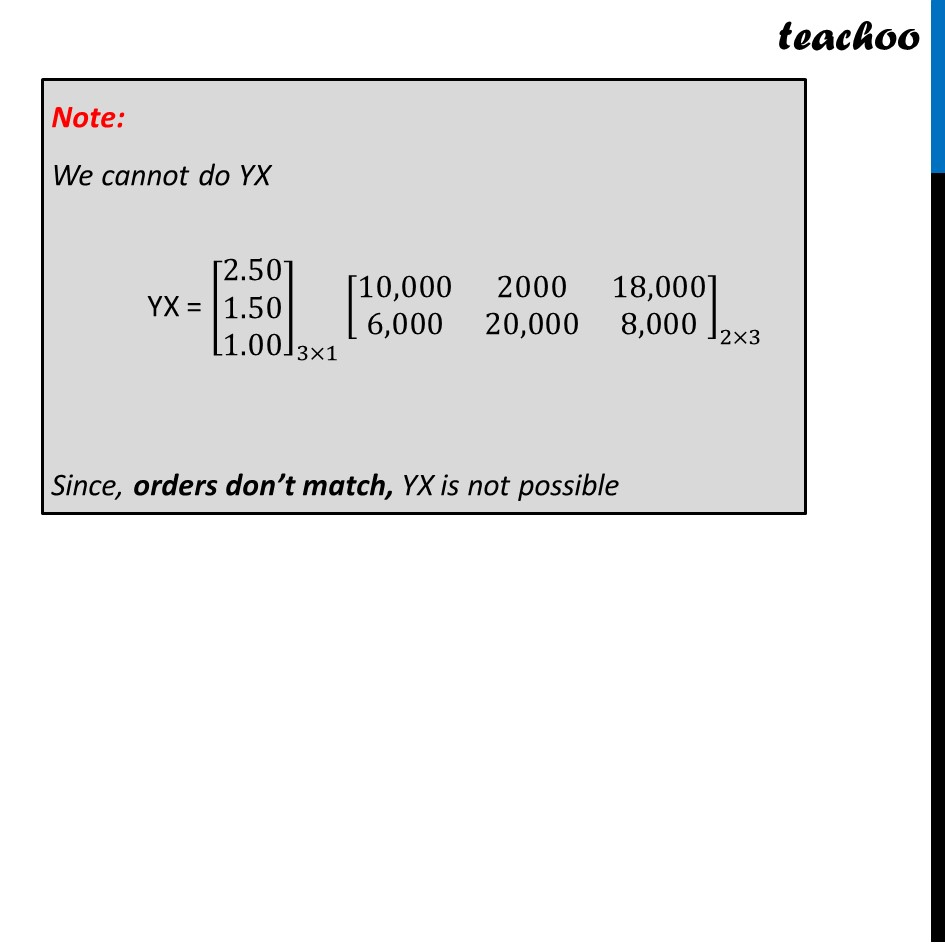## (a) Rs. 35,000 (b) Rs. 53,000 (c) Rs. 50,300 (d) Rs. 30,500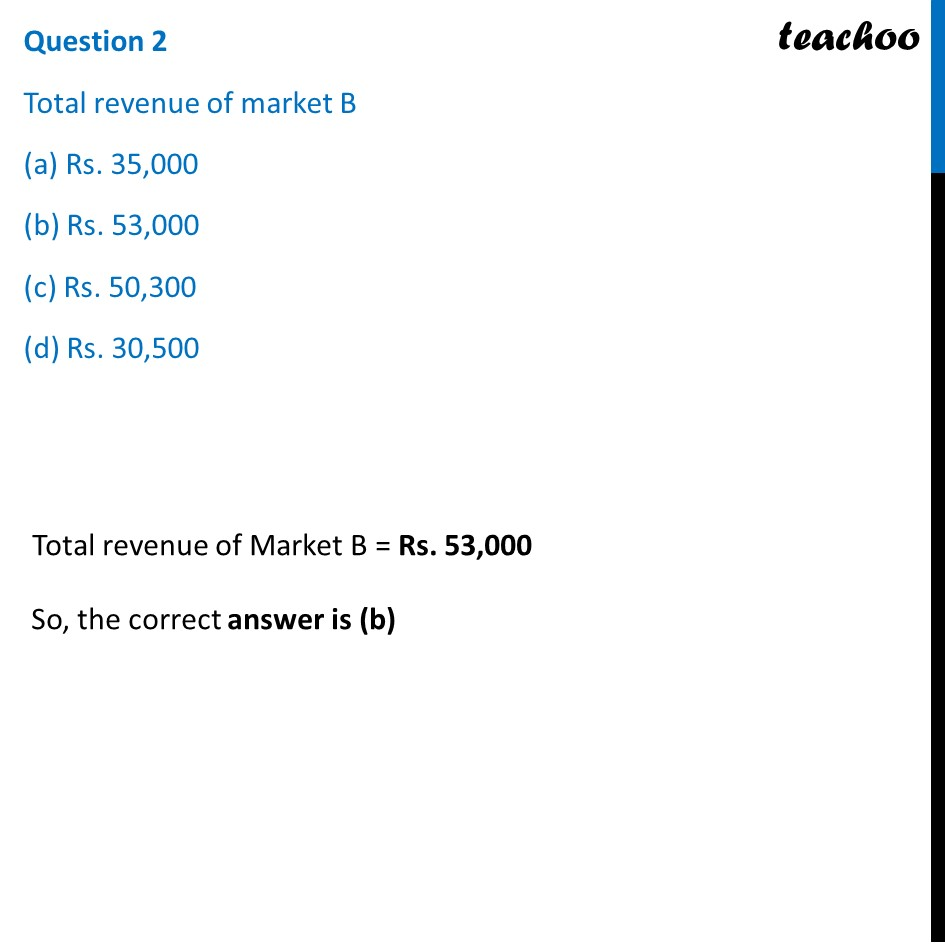## (a) Rs. 13,000 (b) Rs. 30,100 (c) Rs. 10,300 (d) Rs. 31,000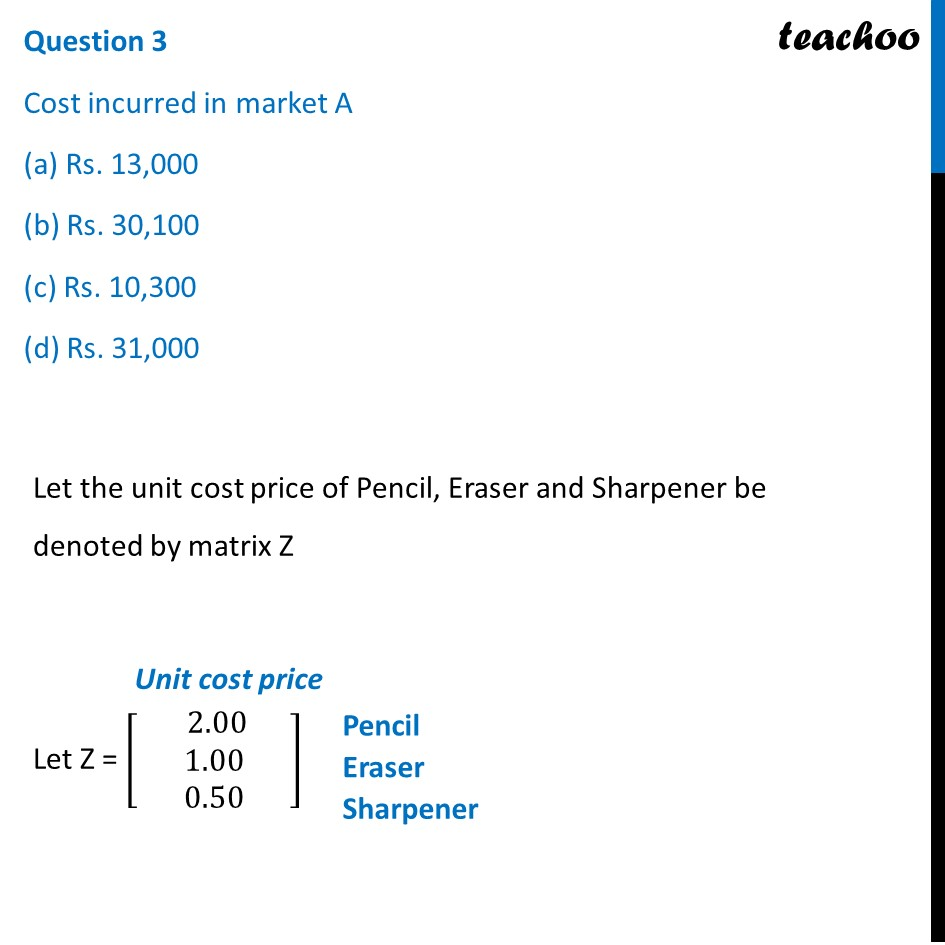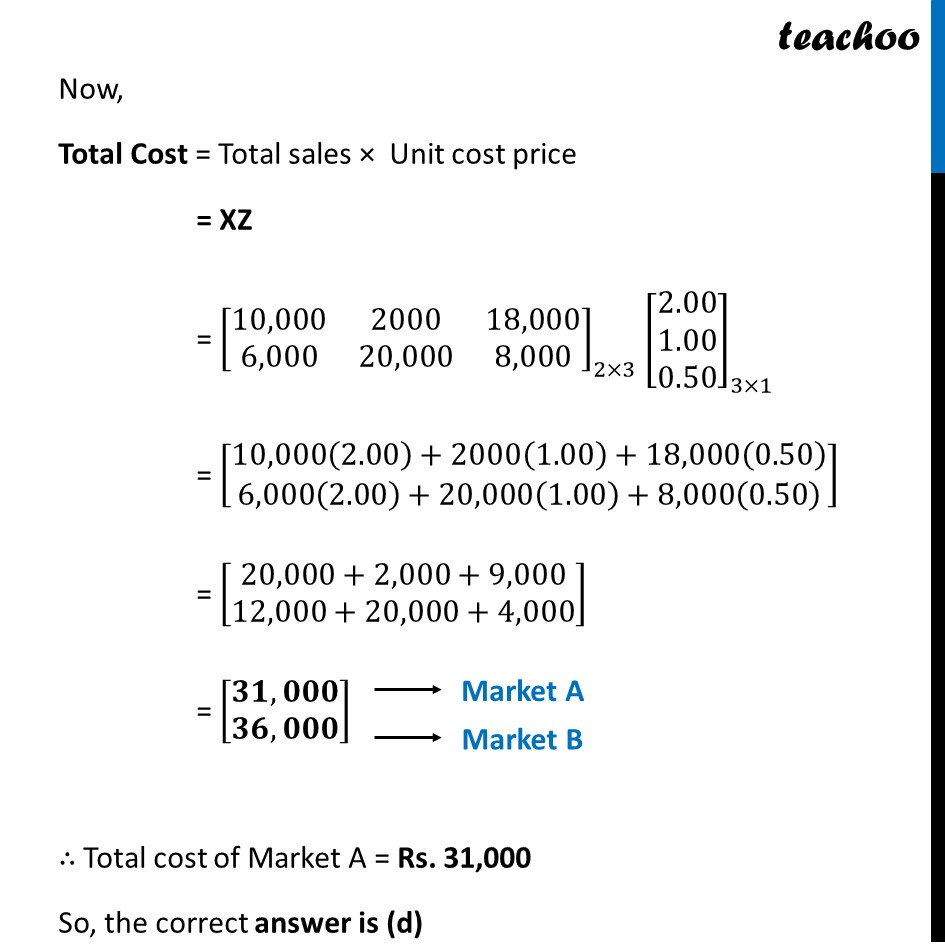## (a) (Rs. 15,000, Rs. 17,000) (b) (Rs. 17,000, Rs. 15,000) (c) (Rs. 51,000, Rs. 71,000) (d) ( Rs. 10,000, Rs. 20,000)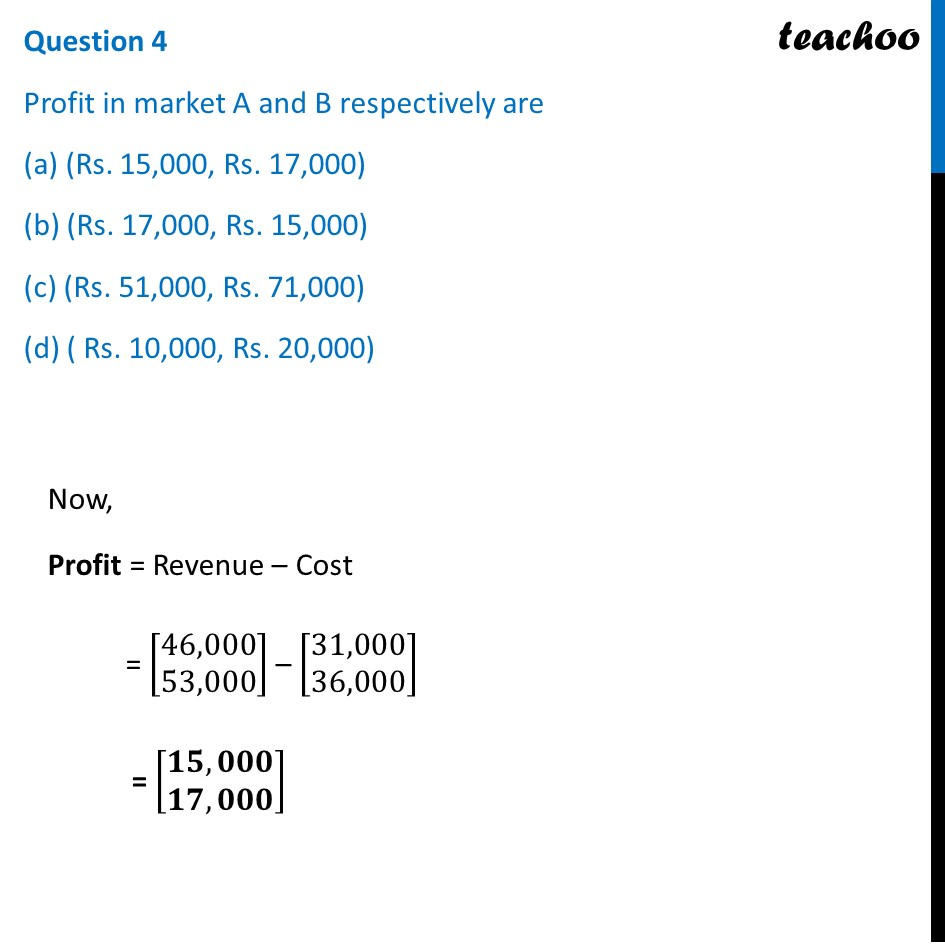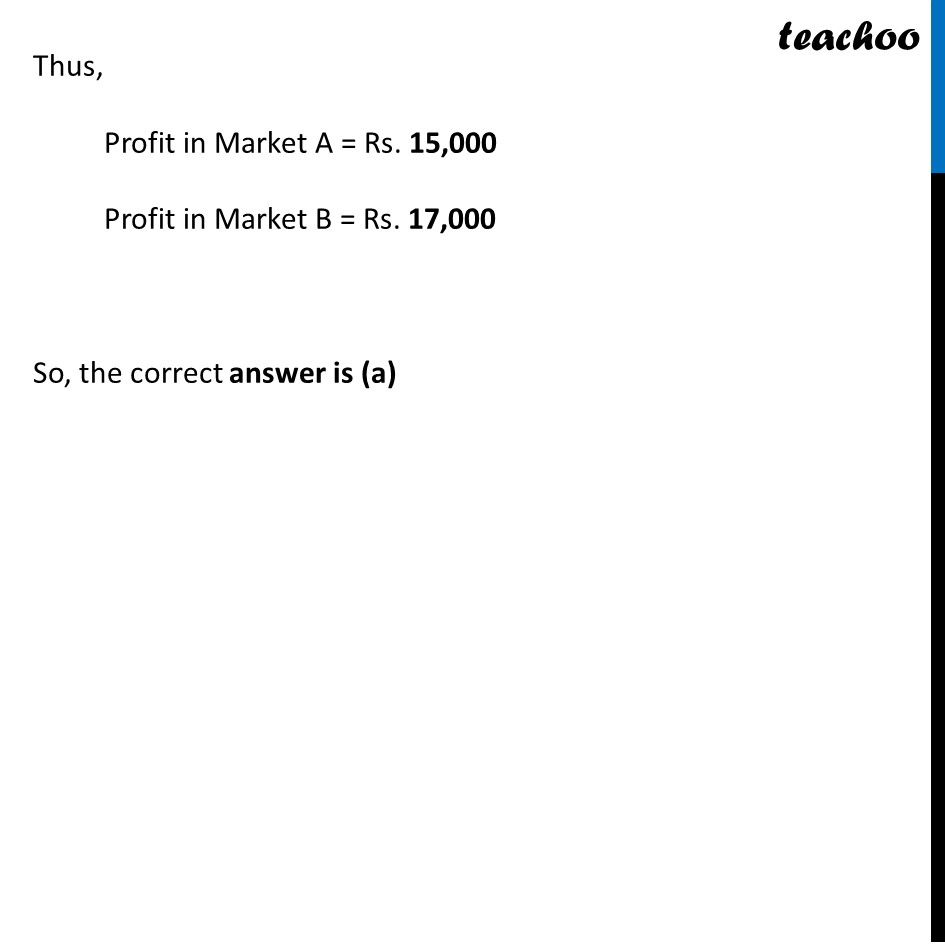## (a) Rs. 23,000 (b) Rs. 20,300 (c) Rs. 32,000 (d) Rs. 30,200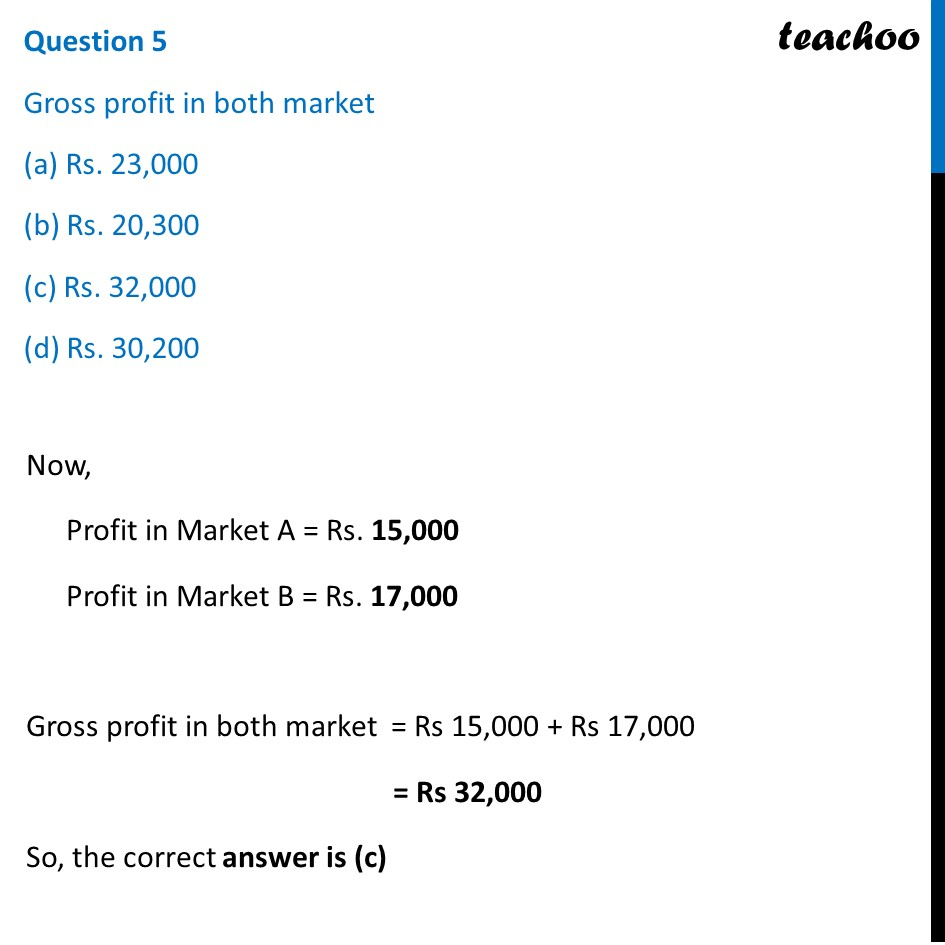Learn in your speed, with individual attention - Teachoo Maths 1-on-1 Class

### Transcript

Question A manufacture produces three stationery products Pencil, Eraser and Sharpener which he sells in two markets. Annual sales are indicated below If the unit Sale price of Pencil, Eraser and Sharpener are Rs. 2.50, Rs. 1.50 and Rs. 1.00 respectively, and unit cost of the above three commodities are Rs. 2.00, Rs. 1.00 and Rs. 0.50 respectively, then, Based on the above information answer the following: Question 1 Total revenue of market A (a) Rs. 64,000 (b) Rs. 60,400 (c) Rs. 46,000 (d) Rs. 40,600Let the sales of Pencil, Eraser and Sharpener be denoted by matrix X X = [■8(10,000&2,000&18,[email protected],000&20,000&8,000)] Let the unit sale price of Pencil, Eraser and Sharpener be denoted by matrix Y Let Y = [■8( 2.50 @[email protected])] Now, Total Revenue = Total sales × Unit sales price = XY = [■8(10,000&2,000&18,[email protected],000&20,000&8,000)]_(2×3) [■8([email protected]@1.00)]_(3×1) = [■8(10,000(2.50)+2,000(1.50)+18,000(1)@6,000(2.50)+20,000(1.50)+8,000(1) )] = [■8(25,000+3,000+18,[email protected],000+30,000+8,000)] = [■8(46,[email protected],000)] Total Revenue = [■8(46,[email protected],000)] Hence, Total revenue of Market A = Rs. 46,000 Total revenue of Market B = Rs. 53,000 So, the correct answer is (c) Note: We cannot do YX YX = [■8([email protected]@1.00)]_(3×1) [■8(10,000&2000&18,[email protected],000&20,000&8,000)]_(2×3) Since, orders don’t match, YX is not possible Question 2 Total revenue of market B (a) Rs. 35,000 (b) Rs. 53,000 (c) Rs. 50,300 (d) Rs. 30,500 Total revenue of Market B = Rs. 53,000 So, the correct answer is (b) Question 3 Cost incurred in market A (a) Rs. 13,000 (b) Rs. 30,100 (c) Rs. 10,300 (d) Rs. 31,000 Let the unit cost price of Pencil, Eraser and Sharpener be denoted by matrix Z Let Z = [■8( 2.00 @[email protected])] Now, Total Cost = Total sales × Unit cost price = XZ = [■8(10,000&2000&18,[email protected],000&20,000&8,000)]_(2×3) [■8([email protected]@0.50)]_(3×1) = [■8(10,000(2.00)+2000(1.00)+18,000(0.50)@6,000(2.00)+20,000(1.00)+8,000(0.50) )] = [■8(20,000+2,000+9,[email protected],000+20,000+4,000)] = [■8(𝟑𝟏,𝟎𝟎𝟎@𝟑𝟔,𝟎𝟎𝟎)] ∴ Total cost of Market A = Rs. 31,000 So, the correct answer is (d) Question 4 Profit in market A and B respectively are (a) (Rs. 15,000, Rs. 17,000) (b) (Rs. 17,000, Rs. 15,000) (c) (Rs. 51,000, Rs. 71,000) (d) ( Rs. 10,000, Rs. 20,000) Now, Profit = Revenue – Cost = [■8(46,[email protected],000)] – [■8(31,[email protected],000)] = [■8(𝟏𝟓,𝟎𝟎𝟎@𝟏𝟕,𝟎𝟎𝟎)] Thus, Profit in Market A = Rs. 15,000 Profit in Market B = Rs. 17,000 So, the correct answer is (a) Question 5 Gross profit in both market (a) Rs. 23,000 (b) Rs. 20,300 (c) Rs. 32,000 (d) Rs. 30,200 Now, Profit in Market A = Rs. 15,000 Profit in Market B = Rs. 17,000 Gross profit in both market = Rs 15,000 + Rs 17,000 = Rs 32,000 So, the correct answer is (c)